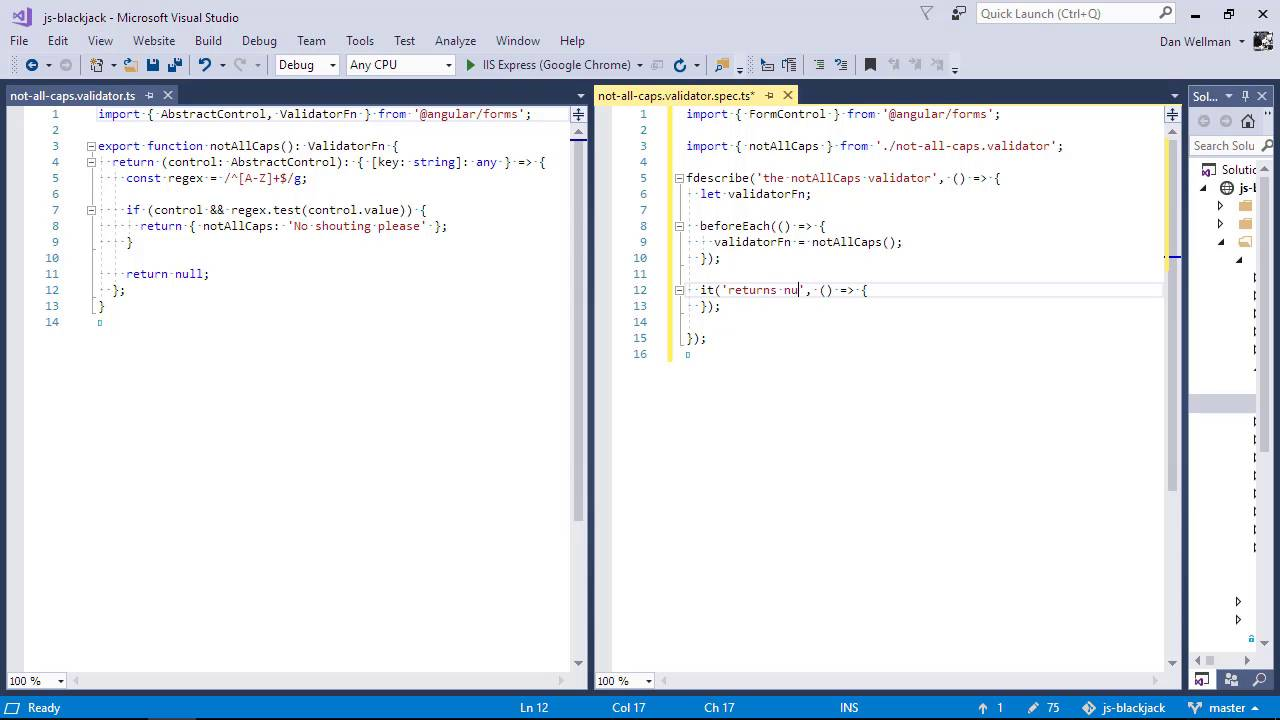Lessons: 67Length: 8.9 hours• Overview
• Transcript

# 8.8 Unit Testing Validators

In this lesson I'll show you how to test a custom validator.

## 10.Conclusion1 lesson, 01:32

### 8.8 Unit Testing Validators

Hi Folks. In this lesson we can take a look at unit testing validators. Validators are another generally small self-contained bit of an angular application, usually with a single purpose. This makes them very easy to test. We do have a validator that we added to the application, the not all caps validator, which validates the value of the form control is not entirely uppercase. We created this file ourselves manually, so we'll need to create the spec file ourselves, manually, too. Let's do that first of all. So we have an _validators directory. This contains the validator. So this is where we'll be adding the spec file. So let's deal with the imports. First of all, we'll need to bring in the formControl class from angular, because the validator validates a form control. And we'll also need to import the validator itself so that we can test it. So now let's add an outer describe. And we've fdescribed it, so that only the tests inside this describe method will run. The validator itself is a function, but it doesn't actually return a value. Instead, it returns another function, and it is this function that is invoked to perform the actual validation. We'll need to invoke the function and keep a reference to the return function so that we can invoke it in our tests. We should probably do this in a beforeEach. Okay, so now let's add a test. So, what does the validator actually do? Well, let's test the null return first of all, because again that's the esiest thing to test. So for this test, we're going to need to create a new form control instance and set its value to a lowercase string. So we create a new FormControl instance. We use the setValue method to set it's value to a lowercase string, we then pass this control to the function returned by the validator, and we then assert that the result is null. So let's check whether the test runs and passes, and we can see that it does. So now we need to test the opposite condition. The validator function should return an object containing an error message if the value of the control is entirely uppercase So this time we set the value of the control to an uppercase string, and this time in the assertion we are checking that the result equals an object that has a key called notAllCaps, and that the value of that key is a string that says 'No shouting please'. So let's go back to the browser. And we can see that the second test is passing as well. So in this lesson, we saw how easy it is to unit test validators in angular. Validators are usually small and self-contained, and this makes them very easy to test. Validators do generally follow a very similar pattern, returning a function, which actually performs the validation, when it's passed to form control. We saw that to test a validator we just need to get a reference to the function it returns, invoke the function with a form control, and then check the value that gets returned by the validator function. Thanks for watching.

Back to the top